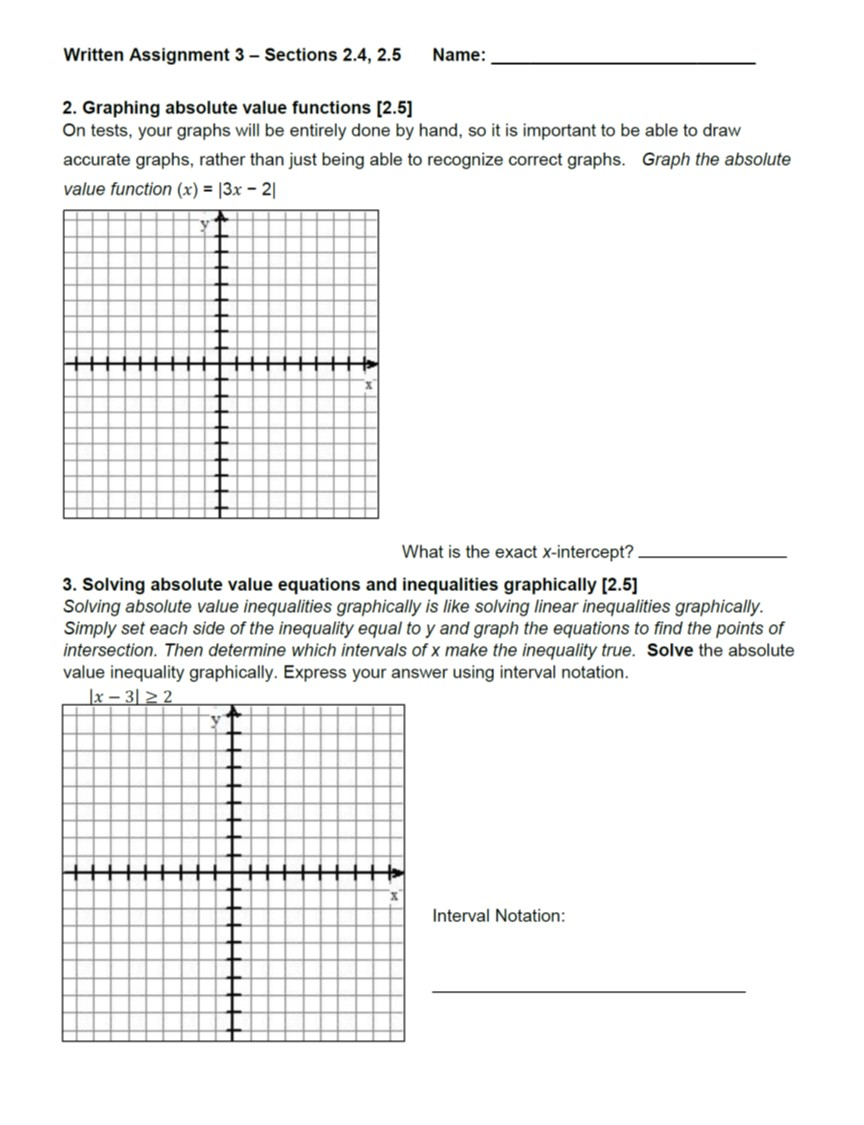# Written Assignment 3 Sections 2.4, 2.5 Name: 2. Graphing absolute value functions [2.5 On tests, your graphs will be entirely done by hand, so it is important to be able to draw accurate graphs, rather than just being able to recognize correct graphs. Graph the absolute value function (x)|3x - 2 What is the exact X-intercept? 3. Solving absolute value equations and inequalities graphically [2.5) Solving absolute value inequalities graphically is like solving linear inequalities graphically Simply set each side of the inequality equal to y and graph the equations to find the points of intersection. Then determine which intervals of x make the inequality true. Solve the absolute value inequality graphically. Express your answer using interval notation. Interval Notation

Question

Dears,
Thankshelp_outlineImage TranscriptioncloseWritten Assignment 3 Sections 2.4, 2.5 Name: 2. Graphing absolute value functions [2.5 On tests, your graphs will be entirely done by hand, so it is important to be able to draw accurate graphs, rather than just being able to recognize correct graphs. Graph the absolute value function (x)|3x - 2 What is the exact X-intercept? 3. Solving absolute value equations and inequalities graphically [2.5) Solving absolute value inequalities graphically is like solving linear inequalities graphically Simply set each side of the inequality equal to y and graph the equations to find the points of intersection. Then determine which intervals of x make the inequality true. Solve the absolute value inequality graphically. Express your answer using interval notation. Interval Notation fullscreen